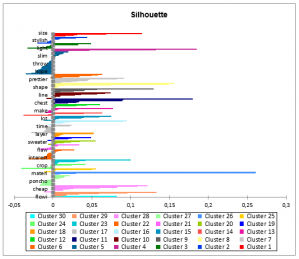# Fuzzy k-means clustering

Use Fuzzy k-means clustering to create homogeneous groups of objects described by a set of quantitative variables.Fuzzy clustering is used to create clusters with unclear borders either because they are to close or even overlap each other. This method was introduced in 1973 by Dunn and Bezdek in 1981. It can highlight sub-clusters and even predict an estimation of the right number of clusters by processing the data with a high number of clusters. Fuzzy k-means is a generalization of the classical k-means.

## Fuzzy k-means clustering options within XLSTAT

### Dissimilarity Index and Clustering Criterion

Several dissimilarity indexes may be used to reach a solution. XLSTAT offers three distances detailed by Chuanren Liu, Tianming Huy, Yong Gez and Hui Xiongx :

• Cosine Dissimilarity: The cosine dissimilarity is the distance which characterizes the spherical k-means and is based on the cosine of the angle between two observations. The wider the angle, the more the cosine dissimilarity will be close to 1, with 1 being an angle of 90° meaning no variables are shared between the observations. In case of textual analysis where the scaling effect has to be small, the cosine dissimilarity is recommended.

• Jaccard Dissimilarity: This distance is based on the extended Jaccard index. The basic Jaccard index computes the binary intersection domain between two binary vectors over the binary union between these observations. The extended Jaccard index does the same thing but considers the values of the vectors as weights. In order to optimize the computation, we base the extended Jaccard index on the cosine similarity.

• Euclidean distance: The Euclidean distance is commonly used in statistical analysis and produces, in most cases, decent results. But, keep in mind that is due to the optimization process, concerning sparse data the other two distances are recommended.

### The clustering criterion QQ (or objective function)

The clustering criterion QQ (or objective function) is computed depending on the choice of clustering distance : for the Euclidean distance three choices are available (Trace(W), Determinant(W), Wilks' Lambda) while for the Jaccard index we use the Trace(W)and for Cosine dissimilarity it is the sum of distances between each observation and centers weighted by μ and m.

### Type of clustering

Hard: Choose this option to compute hard k-means algorithm.

Fuzzy: Choose this option to compute fuzzy k-means algorithm. The default coefficient of fuzziness is 1,05.

## Fuzzy k-means clustering results within XLSTAT

### Global results

Summary table: Activate this option to display the summary of each clustering. This includes the number of clusters and iterations, the clustering criterion, the within-class and between-class sum of squares and the mean width of the silhouette.

Descriptive statistics: Activate this option to display descriptive statistics for the selected variables.

Cluster size: Activate this option to display the number of observations for each cluster.

### Results by class

Centers: Activate this option to display the cluster coordinates.

Central objects: Activate this option to display the coordinates of the nearest observation to the centroid for each class.

Cluster Summary: Activate this option to display the characteristics of each cluster in this partition
(within-class variance, mean, maximum and minimum distances from the cluster center) and all the observations in the clusters.

Most present variables: Activate this option to display the most present variables of each cluster. The default number of words displayed is 10.

Memberships: Activate this option to display the cluster associated with each observation and the distance between these two.

Membershipprobabilities: Activate this option to display the membership probabilities \mu_{i,j}μi,j for each observation (Only available with Fuzzy clustering)

### Charts:

Evolution of the criterion: If you choose to do the clustering between two numbers of clusters, XLSTAT displays the criterion for each partition. The higher the number of clusters and the lower this criterion will be. If there is no particular structure in the dataset, the criterion will decrease steadily but if there is a structure inside the dataset, an elbow might appear on the chart at the right number of clusters.

Profile plot: This chart allows to compare the means of the different clusters that have been created.

Cluster Size: This chart represents the number of observation of each cluster.

Silhouette: Activate this option to plot the silhouette of the partition. For each observation, a fitness coefficient between -1 and 1 will be computed, 1 being the perfect fit and negative values being a bad partition. All these fitness coefficients form the silhouette of the partition. The fitness coefficients are computed as follow :### analyze your data with xlstat

14-day free trial

Included in

Related features